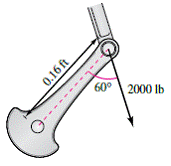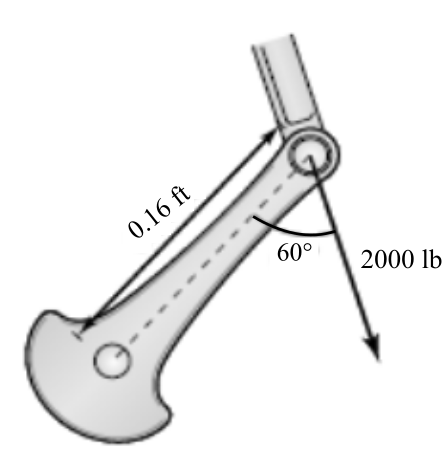Chapter 11.4, Problem 30E

Chapter
Section
Textbook Problem

# Torque Both the magnitude and the direction of the force on a crankshaft change as the crankshaft rotates. Find the torque on the crankshaft using the position and data shown in the figure.To determine

To calculate: The torque acting on the crankshaft using the position and data is shown in the figure where force acting downward as 2000 lb and length of crank is 0.16 ft.Explanation

Given:

Both the magnitude and the direction of force as the crankshaft rotates have been shown in the figure given below where force acting downward as 2000 lb and length of crank is 0.16 ft.

Formula used:

The torque at a point is given by,

t=F×r=Frsinθ

Calculation:

Firstly, sketch the free body diagram which is shown below:

So, force is in the negative Z direction.

Here,

F=2000lb and r=0

### Still sussing out bartleby?

Check out a sample textbook solution.

See a sample solution

#### The Solution to Your Study Problems

Bartleby provides explanations to thousands of textbook problems written by our experts, many with advanced degrees!

Get Started